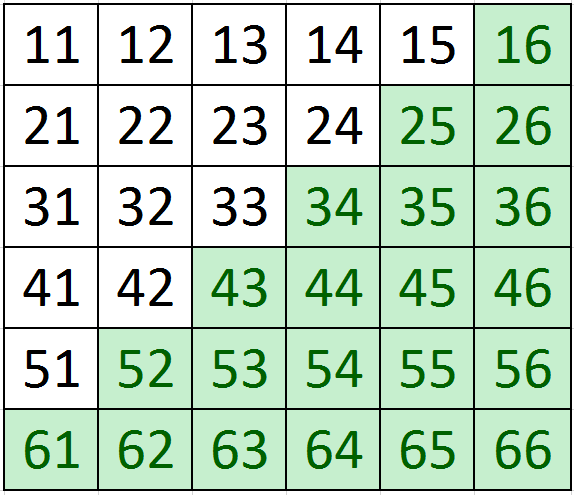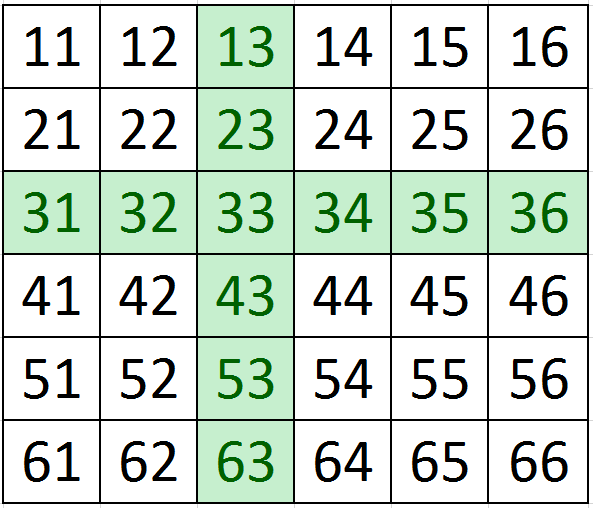0
2845

# Probability Questions for RRB NTPC PDF

Download RRB NTPC Probability Questions and Answers PDF. Top 15 RRB NTPC Probability questions based on asked questions in previous exam papers very important for the Railway NTPC exam.

Question 1: The Indian cricket team is to be selected out of fifteen players five of them are bowlers In how many ways the team can be selected so that the team contains at least three bowlers ?

a) 1260

b) 1620

c) 1250

d) 1200

Question 2: How many different words can be made from the five vowels such that exactly one letter is repeated?

a) 360

b) 720

c) 1440

d) 1800

Question 3: If three coins are tossed, what is the probability that atleast 2 heads occur?

a) 0.5

b) 0.25

c) 0.2

d) 0.1

Question 4: Find the probability that at least 3 heads appear when 4 unbiased coins are tossed.

a) $\frac{11}{16}$

b) $\frac{5}{16}$

c) $\frac{3}{8}$

d) $\frac{5}{8}$

Question 5: Two dice are thrown and the numbers that appear on them are a and b respectively. Find the probability that the sum of a and b is 8.

a) $\frac{5}{6}$

b) $\frac{1}{6}$

c) $\frac{5}{36}$

d) $\frac{31}{36}$

Question 6: If I pick up a card from a well-shuffled pack of cards, What will be the probability of getting an ace?

a) 1/20

b) 1/15

c) 1/13

d) 1/16

Question 7: What is the probability of getting 3 face cards if three cards are drawn from a set of 52 playing cards?

a) $\frac{11}{1105}$

b) $\frac{7}{1105}$

c) $\frac{17}{1105}$

d) $\frac{19}{1105}$

Question 8: In how many ways can we arrange the letters of the COMMIT so that the vowels are at the extreme ends?

a) 14

b) 24

c) 6!/2!

d) 36

Question 9: In a wooden box, there are four red and four black balls. If two balls are taken out simultaneously at random, then the probability of both balls being red is:

a) $\frac{1}{2}$

b) $\frac{1}{4}$

c) $\frac{3}{8}$

d) $\frac{3}{14}$

Question 10: A complete cycle of a traffic lights takes 60 seconds. During each cycle the light is green for 25 seconds, yellow for 5 seconds and red for 30 seconds. At a randomly chosen time, the probability that the light will not be green is:

a) $\frac{1}{3}$

b) $\frac{1}{4}$

c) $\frac{5}{12}$

d) $\frac{7}{12}$

Question 11: A fair die is rolled twice. What is the probability that the sum of the two outcomes is more than 6?

a) $\frac{2}{9}$

b) $\frac{1}{4}$

c) $\frac{7}{12}$

d) $\frac{4}{9}$

Question 12: Let P (n) be the probability that the outcome is ‘n’ when a die is rolled. A biased die is such that,
– P (1) = P (3) = P (5) = x
– P (2) = P (4) = P (6) = y.
– y = 2x.
What is the probability that the outcome is a prime when the die is rolled?

a) $\frac{4}{9}$

b) $\frac{5}{9}$

c) $\frac{1}{2}$

d) $\frac{1}{3}$

Question 13: Two fair dice are rolled simultaneously. What is the probability that atleast one of the dice shows up a three?

a) $\frac{5}{6}$

b) $\frac{11}{36}$

c) $\frac{1}{2}$

d) $\frac{25}{36}$

Question 14: Rajat and Piyush were playing Scrabbles. Piyush made the word ‘BUMFUZZLED’. Rajat took 5 letters from this at random and arranged them randomly. What is the probability that he made the word ‘FUMED’?

a) $\frac{1}{126}$

b) $\frac{1}{3024}$

c) $\frac{5}{9072}$

d) None of these

Question 15: A real number $k$ is chosen from 0 to 15. What is the probability that $k^2$ $\geq$49?

a) 8/15

b) 1/2

c) 7/16

d) none of these

To select a team of 11, there can be 5 bowlers and 6 batsmen or 4 bowlers and 7 batsmen or 3 bowlers and 8 batsmen. This can be done in $C_6^{10} + C_4^5 \times C_7^{10} + C_3^5 \times C_8^{10}$

= 1260

In total, there are 6 letters of which one is repeated.
Number of ways of arranging 6 letters is 6! = 720

One vowel out of 5 can be selected in 5 ways.
One repitition => 5*720/2! = 1800

The total number of possibilities are TTT, TTH, THT, HTT, THH, HTH, HHT and HHH.
We need to select those cases in which there are at least 2 Hs.
There are 4 such cases out of 8.
=> Probability = 4/8 = 0.5

Favourable cases are HHHT, HHTH, HTHH, THHH and HHHH => 5
Total number of cases are 2*2*2*2 = 16
Probability = $\frac{5}{16}$

Number of ways such that sum is 8 => (2,6), (3,5), (4,4), (5,3), (6,2)
=> 5 possible cases.
Total number of cases = 6*6 = 36
Probability = $\frac{5}{36}$

As there are a total of 4 ace cards out of 52 cards hence probability will be = 4/52 = 1/13

There are 12 face cards in each deck : 4 Kings, 4 Queens and 4 Jacks. We can select 3 out of these in $^{12} C _3$ ways. We can select 3 cards in $^{52} C _3$ ways. Hence, probability = $^{12} C _3$ / $^{52} C _3$ = 12*11*10/52*51*50 = 11/1105.

The vowels would be at either ends. The remaining letters can be arranged in 4!/2! = 12 ways. The vowels can be arranged among themselves in 2! =2 ways. Total number of ways = 12*2=24 ways.

Probability of both balls being red=$\frac{4}{8}$×$\frac{3}{7}$=3/14

probability that the light is not green=probablity that the light is green or red=(30 +5)/60=7/12

The favourable cases are when the sum is 7, 8, 9, 10, 11 and 12.
We can use the 6×6 matrix to find out the number of ways in which the sum can be 7, 8, 9, 10, 11 and 12.
These are as highlighted in green.Thus the number of ways = 6+5+4+3+2+1 = 21.
Probability = 21/36 = 7/12.

We know that,
P(1)+P(2)+P(3)+P(4)+P(5)+P(6) = 1
=> 3x+3y = 1
=> 3x+6x = 1
=> x = 1/9
Thus,
P (1) = P (3) = P (5) = 1/9
P (2) = P (4) = P (6) = 2/9
The favourable outcomes are 2, 3 and 5.
Required Probability = P(2) +P(3) +P(5) = 4/9.

We can use the 6×6 matrix to find out the number of ways in which atleast one of the dice shows up a three.
The favourable cells are as highlighted in green.Thus the number of favourable ways = 11.
Probability = 11/36.

The probability that he took the letters- F, U, M, E, and D is $\frac{2}{^{10}C_{5}}=\frac{2}{252}=\frac{1}{126}$. [2 Us are available]
After selecting the letters, the probability that he arranged them in this particular order is $\frac{1}{5!}$
So, the probability that he made ‘FUMED’ is $\frac{1}{126} \times \frac{1}{5!} = \frac{1}{15120}$
$k^2 \geq$ 7 only from 7 to 15. From 7 – 15, there are 8 equal ranges.